### Prime Walk

July 12, 2021

Humans try to find patterns in things. As a child in school, I'm fairly certain that's the way that I learned mathematics. As a computer programmer, I find that discovering one good example on the Internet is better than a textbook chapter. As in most human things, the concept can be taken too far. That's why people try to find hidden messages in the Bible. I vaguely remember the plot of a 60s television show that has a scientist and his followers retreat to a cave since they are sure that a nuclear war would occur at a specific time. The time was related to the rising of the constellation, Boötes, simply because a Russiann scientist had misspelled boots as bootes in a letter.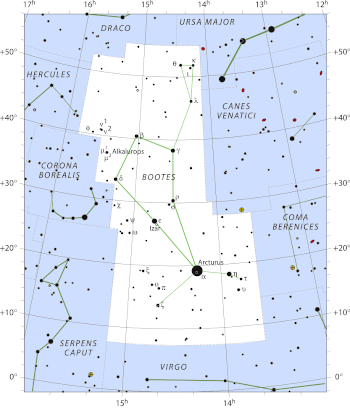The constellation, Boötes (The Plowman), is not well known, since it is not among the twelve constellations of the Zodiac. However, it is among the 48 constellations described by Ptolemy (c.100-c.170), and it is one of the 88 modern constellations.

Arcturus (α-Boötes), which is 36.7 light-years from Earth, is the fourth-brightest star in the night sky (apparent magnitude, -0.05)

(Modified Wikimedia Commons image by Roger Sinnott & Rick Fienberg/the IAU/Sky & Telescope magazine. Click for larger image.)

In 1932, American herpetologist, Laurence Monroe Klauber (1883-1968) presented a paper to the Mathematical Association of America in which he showed the geometric regularity in the distribution of the prime numbers when the natural numbers were arranged in a triangle (see figure).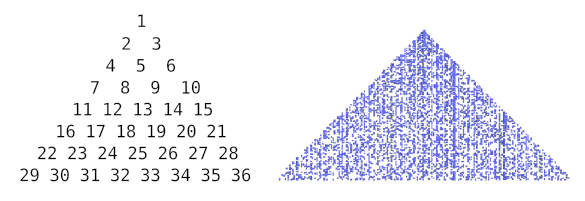Klauber's triangular representation of the prime numbers. The plot on the right, generated using the Python program in ref. 1, highlights the primes as colored points. (Klauber.py source here. Click for larger image.)

Klauber noted that this phenomenon was related to prime-generating polynomials, such as the one devised by Euler. In a 1772 letter to Johann Bernoulli, Euler mentioned that the function,
f(n) = n2 + n + 41
has prime values for 0 ≤ n ≤ 39.[2-3] This function fails at n = 40, which gives f(n) = 1681, but it gives primes with a high frequency for larger n.

In 1963, Polish-American physicist, Stanisław Ulam (1909-1984), independently discovered a similar phenomenon on a square lattice of numbers that's now known as the Ulam spiral (see figure). Ulam made this discovery while doodling during presentation of "a long and very boring paper" at a scientific conference, something that many scientists have experienced.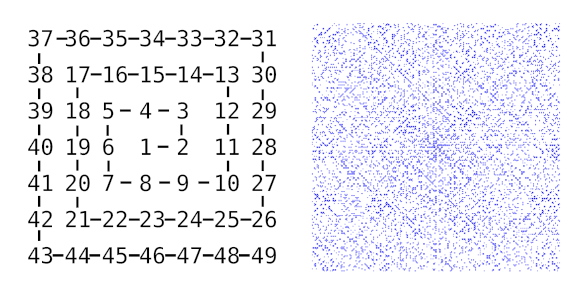The Ulam spiral representation of the prime numbers. The plot was generated using the Python program in ref. 4. (Ulam.py source here. Click for larger image.)

The Ulam spiral was popularized by Martin Gardner in his March, 1964, Mathematical Games column in Scientific American. Ulam, who had an early interest in computers, and his colleagues used the Los Alamos MANIAC II to produce an spiral of about 100,000 points, and they investigated some prime-rich and prime-poor lines.

An international team of scientists was inspired by the Ulam spiral to create a novel random walk in two-dimensions that they call a prime walk. The team had members from the Czech Technical University in Prague (Prague, Czech Republic), the Universidade de São Paulo (São Paulo, Brazil), the Universidad del País Vasco (Leioa, Spain), the National Kapodistrian University of Athens (Athens, Greece), and the University of Iceland (Reykjavík, Iceland). Their study was published recently on arXiv, and later in Physical Review E.

A random walk on a square lattice can be created by having a point trace a path by moving randomly up, down, left, or right. The prime walk has instead the following rules based on the idea that (aside from the prime numbers 2 and 5) the last digits of prime numbers are 1, 3, 7 and 9:
• The starting point at (0, 0) is given the value N = 1.

• At the current point (x, y), find N + 1.

• If N + 1 is not a prime, don't move. If it is a prime, assign N + 1 to a new position, as follows.

• If N + 1 is a prime and its last digit is 1, move up; i.e., (x, y) -> (x, y + 1).

• If N + 1 is a prime and its last digit is 3, move down; i.e., (x, y) -> (x, y − 1).

• If N + 1 is a prime and its last digit is 7, move left; i.e., (x, y) -> (x − 1, y).

• If N + 1 is a prime and its last digit is 9, move right; i.e., (x, y) -> (x + 1, y).
A computation can track how many times a lattice point has been visited, allowing the visualization of the prime walk in the following figure.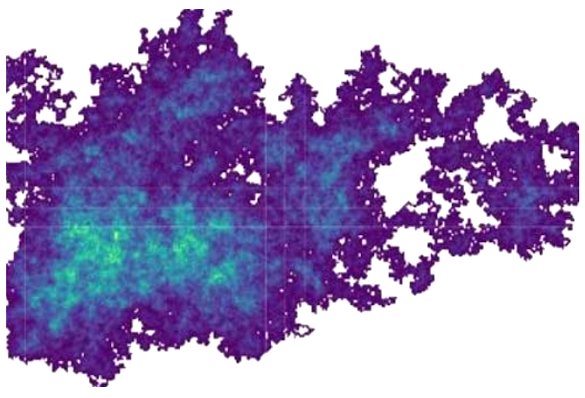Visualization of the prime walk. The shading shows how often a lattice point was visited. (Portion of fig.1 of ref. 5.)

The prime walk has some interesting properties, one of which is that the covered area is 1/10 the number of primes. Furthermore, the first digit of the maximum number of cell visits follows Benford's law (see figure).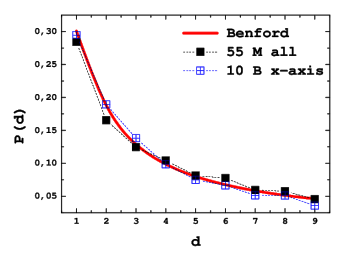Benford's law for the prime walk. This is a histogram of the leading digits of the maximum number of cell visits for walks to length 5.5 x 107 (black squares).

The blue squares are for values on the x-axis for walks up to 109 steps. Benford's law is shown by the red curve.

(Fig. 6 of ref. 5.)

### References:

Linked Keywords: Human; pattern; child; grammar school; mathematics; computer programmer; Internet; textbook; chapter (books); Bible code; hidden messages in the Bible; plot (narrative); 1960s; television program; television show; scientist; cave; nuclear warfare; nuclear war; heliacal rising; rising; constellation; Boötes; Russia; Russian; scientist; boot; letter (message); The Plowman; Zodiac; Ptolemy (c.100-c.170); Arcturus (α-Boötes); light-year; Earth; star; night sky; apparent magnitude; Wikimedia Common; America; American; herpetology; herpetologist; Laurence Monroe Klauber (1883-1968); academic publishing; paper; Mathematical Association of America; geometry; geometric; prime number; natural number; triangle; triangular; visualization (graphics); representation; plot (graphics); Python (programming language); computer program; Klauber.py source; phenomenon; prime-generating polynomial; Leonhard Euler; Johann Bernoulli; function (mathematics); frequency; Poland; Polish; physicist; Stanisław Ulam (1909-1984); square lattice; Ulam spiral; doodle; doodling; presentation; scientific conference; Ulam.py source; popular science; popularize; Martin Gardner; Mathematical Games column; Scientific American; computer; colleague; Los Alamos National Laboratory; MANIAC II; random walk; two-dimensional space; Czech Technical University in Prague (Prague, Czech Republic); Universidade de São Paulo (São Paulo, Brazil); Universidad del País Vasco (Leioa, Spain); National Kapodistrian University of Athens (Athens, Greece); University of Iceland (Reykjavík, Iceland); arXiv; randomness; random; algorithm; rules; numerical digit">digit; computation; Visualization; lattice graph; lattice point; area; Benford's law; histogram.